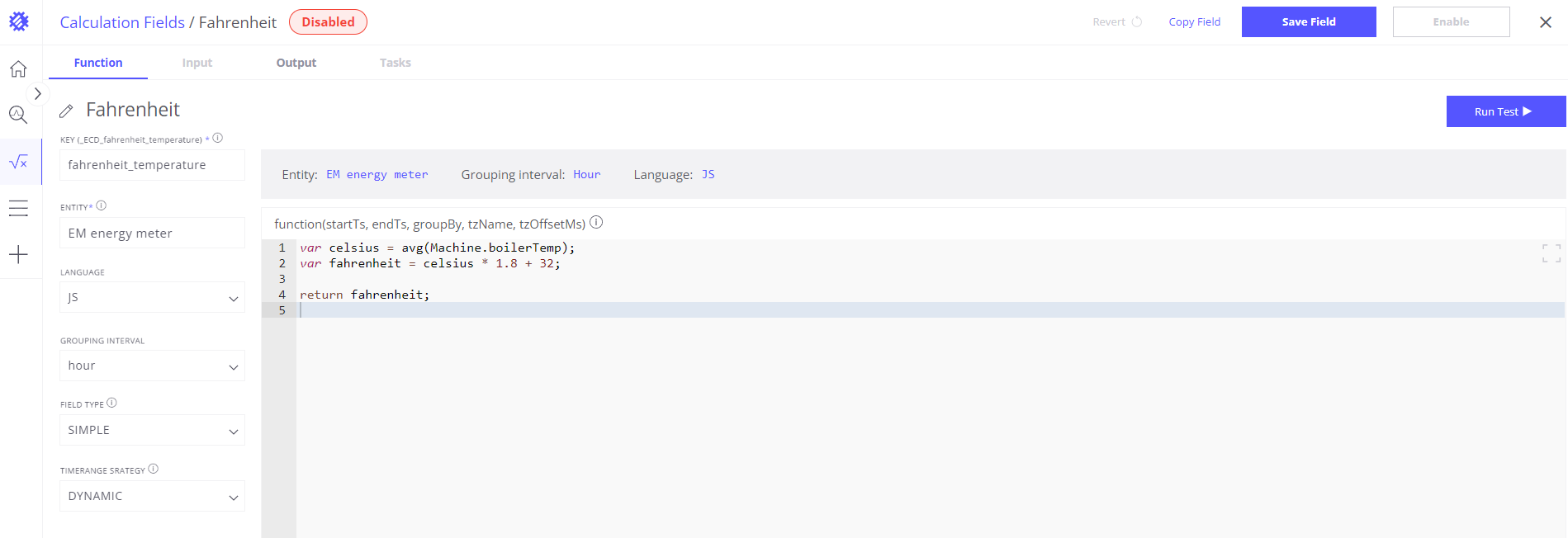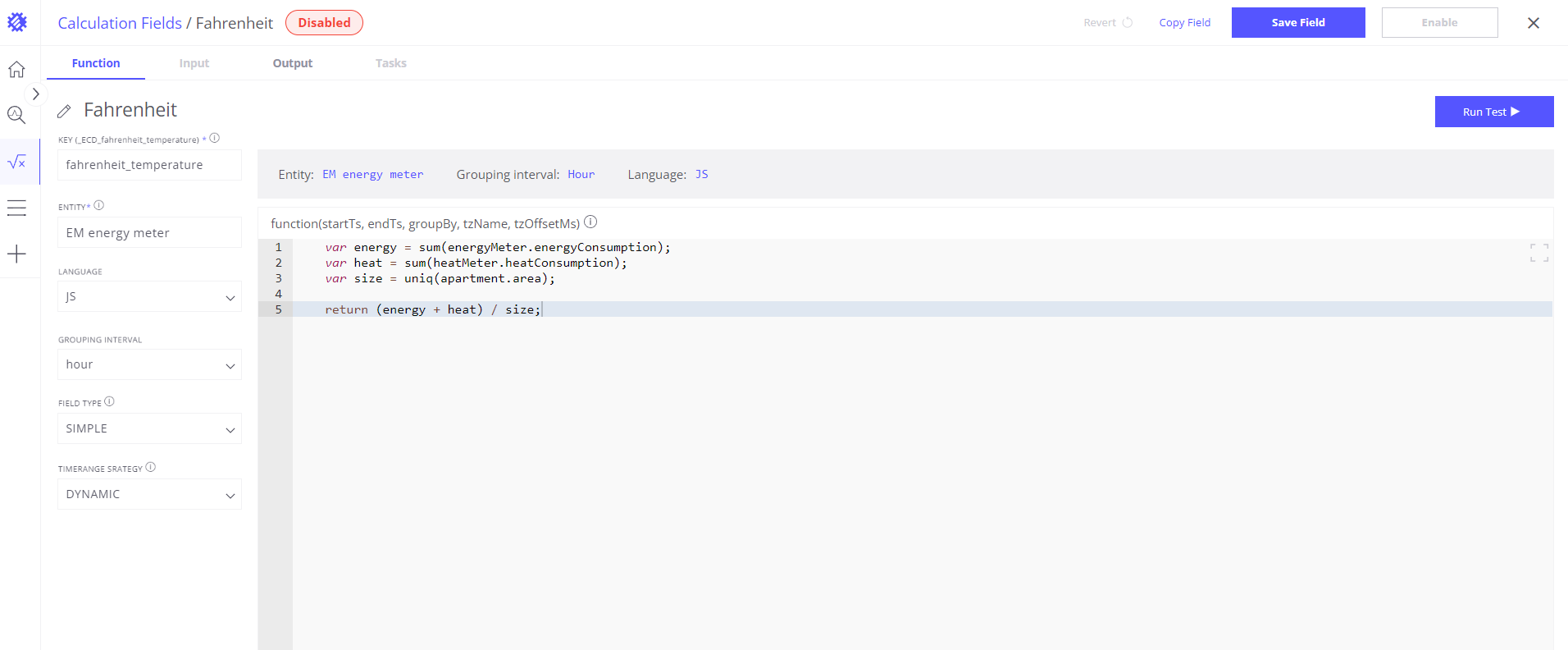Trendz Analytics
Documentation > Calculated Fields > Overview

# Calculated Fields

Calculated fields are one of the most powerful features for KPI monitoring and prediction. Based on the input data, calculated fields allow you to run statistical functions and create new data items by applying calculations. As Trendz Analytics processes the calculations on the fly, no data from ThingsBoard database will be damage. And no additional load will be applied.

## Simple calculation

Let’s assume that sensor submit boiler temperature in Celsius and we want convert it to Fahrenheit:

• Drop Calculated field from left navigation to the Y axis section
• Open field configration and write transformation function## Multiple fields for calculation

In this example we have Apartment asset that has 2 sensors installed - HeatMeter and EnergyMeter. Both sensors submit how much energy was consumed. Also Apartment has area attribute that contains apartment size. We want calculate total energy consumed by HeatMeter and EnergyMeter in Apartment per square meter. Let’s break it to subtasks:

• Get amount of energy consumed by HeatMeter - heatConsumption telemetry
• Get amount of energy consumed by EnergyMeter - energyConsumption telemetry
• Get Appartment size - area attribute
• Sum heatConsumption and energyConsumption
• Devide it by area

For implementing this we need to:

• Drop Calculated field from left navigation to the Y axis section
• Open field configration and write transformation function## Get original field value

Before applying transformation you need to get a reference to the original field value. Here is an example how to do this:

``````1
var temp = avg(Machine.temperature);
``````
• avg() - aggregation function
• Machine - Entity Name (it can be Asset Type or Device Type)
• temperature - Field Name

All 3 parts are required, you can not access original field value without aggregation function.

If original field value is an attribute, entity name or owner name - you should use uniq() aggregation function.

## Supported Aggregation Functions

JSEditor for calculated fields supports following aggregation functions:

• avg()
• sum()
• min()
• max()
• count()
• latest()
• uniq()

Each function allows only 1 argument - reference to the filed on format EntityName.fieldName. For example:

``````1
sum(Machine.temperature)
``````

Aggregation function applied to a grouped dataset. Find more details about Grouping and Aggregation in this article

## Save and reuse calculated fields

Once the calculated field is created you can save it for future reuse by pressing Save Field button under function editor. Current field label would be used as a field name. If a field with such name already exists - the system will overwrite it.

Saved calculated field is only a template. Once it is dropped from the left navigation tree into some axis, a new calculated field created and this field would not be connected with the original template. It means that if you will update field configuration in the future, it will only update a template, but real calculated fields that are added to View configuration are not affected.

## Language and script engine

You can write calculated fields using Javascript or Python. By default, Javascript engine is selected.

• Javascript - Calculated Fields use Javascript as a language for writing transformation function. Inner Engine provide 100% support of ECMAScript 5.1
• Python - Python script engine support python 3.8 and provide full access to python standard libraries. Additionally, you can use following libraries: flask, numpy, statsmodels, pandas, scikit-learn, prophet, seaborn, pmdarima.